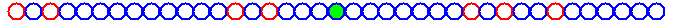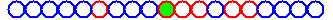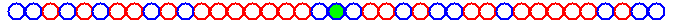###### (probability of having a red coin=20%, probability of going right with red coin=70%, probability of going right with blue coin=48%, boundaries bounce back to zero)

The model is simple. For the motivation behind such a model, scroll down. I will start by explaining the one-dimensional case.

Space: First, I need to explain where this "walk" is happening! For this, imagine a long long straight road (say Broadway!), with a lot of stores, side by side, one after another.

Time: Let's fix a time unit, say an hour. This will mean later that once we arrive at a store, we will stay there for an hour. Then, after the hour has passed, we will move to another store, and so on.

Environment: Imagine we color each store with either red or blue. We then have an environment. Note that a special environment is when all stores are colored with the same color. We call such an environment "simple".

Random Environment: Let us now pick the colors at random. For example, use your favorite coin to pick the color each time. Say, heads for red and tails for blue. Toss this coin once for each store, and color that store accordingly. This is now a random environment.

Of course, the coin doesn't have to be fair, and one color could be preferred to the other! For example, as an extreme case, if the coin is loaded so that you always get heads, then you will have the simple environment consisting of only red stores.

Random Walk in a Random Environment: Once you have created a random environment, grab two coins and color one red and one blue. We will use these two coins to move around as follows:

When you arrive at a store, you stay there for a whole time unit (say an hour). When your time at that store expires, you look at the color of the store. If you are in a red store, then you toss the red coin to decide whether you move to the store on the left (say if you get tails) or on the right (say if you get heads). If you are in a blue store, then toss the blue coin instead, and then move left or right accordingly. You now have a random walk in a random environment.###### (probability of having a red coin=30%, probability of going right with red coin=70%, probability of going right with blue coin=40%, bouncing back in, at boundaries)

Simple Random Walk: If you have a simple environment (i.e. all stores have the same color), then you clearly will always toss the same coin to move around. Such a walk is called a simple random walk. In other words, you choose your favorite coin, and then walk among stores by flipping this coin each time, to decide whether to go left or right.

Multi-Dimensions: Of course, one can do the same thing on a grid (say Manhattan!). Imagine now, you have streets that go east-west and avenues that go south-north. Imagine that at each intersection, you have a corner shop. Color the shops with one of two colors: red or blue, and then use two "4-sided" dice (one red and one blue) to move around. I know dice are usually 6-sided, but you know what I mean! (ignore the numbers 5 and 6, for example, and throw the dice again if you get these..) Now you have a random walk in a two-dimensional random environment.

Now, why stop at two dimensions??!

Speed: One can ask all sorts of questions, concerning random walks. For example, after having walked for a certain number of hours, we can ask ourselves: how far are we now from our starting point? If you calculate this distance, and divide it by the number of hours, that will give you your current speed. One can then ask:

Does the speed approach a certain constant number, as time goes by?

The answer to this question is very easy to see, when we have a simple random walk. For simplicity, let us concentrate on the one-dimensional case. Indeed, let's say that we are using a coin that gives heads with probability p and tails with probability 1-p. To be concrete, let us say it's heads with probability 60% and tails with probability 40%. Then, if we take a large number of steps, we will move, on average, 60% of the time to the right, and 40% of the time to the left. That is, if the number of steps were called n, then we move 0.6n steps to the right and 0.4n steps to the left. So our over all distance would be 0.6n-0.4n, or, in the more general case:
pn-(1-p)n.

So our average speed is then distance over time, which is (pn-(1-pn))/n=p-(1-p). Of course, our position is random and thus so is our speed. But as time goes by (i.e. n becomes large) our speed will get closer and closer to the average speed p-(1-p)=2p-1.

This is quite interesting! Although we are walking in a random manner, we have a NON-random speed after we have walked for a while!!! This is called the Law of Large Numbers.

The above "easy" argument (for a simple random walk) breaks down when we have a non-simple random environment. But the above question about the speed can still be asked.

The answer happens to be yes, once again, in the one and two-dimensional cases. So, as long as we are walking in one or two directions, and even when we have TWO sources of randomness (the random environment, and the random walk itself), we still manage to go with a constant speed!!

The answer happens to also be yes, for "certain" three or more-dimensional environments. It is generally believed to be always the case, no matter what the dimension is, but we (mathematicians) don't have a full proof yet.

There are many other interesting questions, of course. For example, as I mentioned above, the law of large numbers says that the walker will go with a certain constant velocity. But since the walker is moving randomly, after all, there is always a chance that he behaves a-typically, and moves with a velocity that is different from what was expected of him! What are the chances of this happening? This kind of questions can be addressed by Large Deviations theory.

One more example. If, on average, we say we are going with a constant speed (say v), then after time n we are expected to be somewhere around the point nv (time x speed). One can then ask about how far we will actually fluctuate away from this expected position. This is addressed by the Central Limit Theorem. And so on...###### (probability of having a red coin=60%, probability of going right with red coin=70%, probability of going right with blue coin=40%, periodic boundaries)

Motivation: Originally, in its one-dimensional version, this kind of processes appeared as a simple model of DNA transcription.

Imagine an RNA strand as being a long (long enough to be considered "infinite"!) sequence of the letters A, C, G, or T. A DNA is made of two strands each of which is also a sequence of the letters A, C, G, or U. To make a RNA strand, an enzyme goes over one of the strands of the DNA and creates an RNA chain by putting a T where A's appear, an A where U's appear, a G where C's appear, and a C where G's appear. It turns out that when reading the DNA strand, the enzyme does not move along in a linear fashion! It rather performs a random walk along the strand.

Imagine that the DNA is being read from left to right. Then, at any moment in time, what we have is an RNA chain to the left of the enzyme and nothing to the right of it. At this point, the enzyme can choose to either go right and build one more brick of the RNA, or go left and destroy the last brick it just built! Moreover, it turns out that the rates of moving left (destroying) or right (building) depend on the letter that the enzyme is currently seeing, and on the two neighboring letters as well. So these rates really depend on where the enzyme is at, and therefore, the environment in which this random walk is performed is a non-simple environment. Basically, since we have four choices of letters, an environment would consist of choosing, for each site, one of 64 possible coins.

Of course, since the DNA structure is not quite random (unless maybe you are one of the x-men!), the environment is not random. However, the tools that have been developed to study this kind of fixed, but non-simple, environments, happen to be very helpful for the study of one-dimensional random environments, and vice-versa. That's why it is widely agreed upon that random walks in random environments were originally motivated by this kind of questions.

One of the important biological questions was about how fast the DNA strand is replicated (or destroyed!). The techniques of random walks in a one-dimensional random environment provided us with an answer, as was mentioned above.

Of course, one might wonder why the enzyme performs a random walk, instead of just reading the DNA strand from left to right?! It turns out that the random walk helps correcting the possible transcription errors (mutations), since the enzyme then has a chance to revisit (and destroy and then later rebuild) some of the places where it has already been, and where a mutation happened to occur.

In its two-dimensional version, this model can also be used, for example, as a simple model for the spread of radioactive material (diffusion, random walk) underground (random medium).

By now, this model has many applications in the different natural sciences. However, due to the effects of a random medium, the process of random walks in a random environment is rich enough to present a challenging topic even from a pure mathematical point of view.